# 计及多小水电接入配电线路的线损计算方法及降损措施研究Losses Calculation Method and Reduction Measures of Distribution Line with Multi-Small Hydropower

DOI: 10.12677/SG.2020.101001, PDF, HTML, XML, 下载: 144  浏览: 225

Abstract: Line loss is an important index to evaluate the rationality of distribution network planning and operation. With multi-small hydropower units being connected to the distribution network successively, the bidirectional flow characteristics of power flow in the distribution network become increasingly prominent, which increases the difficulty of line loss calculation. In this paper, a line loss calculation model for distribution lines including multi-small hydropower is established, and the analytical formula for line loss of each segment is derived by means of root mean square current method, and the related loss reduction measures are proposed. Combined with specific examples, the line loss values of distribution network in different scenarios are compared, and the effectiveness of loss reduction measures is verified.

1. 引言

2. 线损计算方法

2.1. 均方根电流法

${I}_{\text{rms}}=\sqrt{\underset{0}{\overset{T}{\int }}{I}^{2}\left(t\right)\text{d}t/T}$ (1)

$\Delta A=3R\underset{0}{\overset{T}{\int }}{I}^{2}\left(t\right)\text{d}t×{10}^{-3}$ (2)

$3{I}_{\text{rms}}^{2}=\frac{1}{T}\underset{\text{0}}{\overset{T}{\sum }}\frac{{P}^{2}+{Q}^{2}}{{U}^{2}}$ (3)

${I}_{\text{rms}}=\sqrt{\frac{\underset{\text{0}}{\overset{T}{\sum }}\frac{{P}^{2}+{Q}^{2}}{{U}^{2}}}{3T}}$ (4)

2.2. 等值电阻法

$\Delta P=3\underset{i=1}{\overset{n}{\sum }}{I}_{i}^{2}{R}_{i}=3{I}^{2}{R}_{el}$ (5)

2.3. 最大电流法

$F=\frac{{I}_{\text{rms}}^{2}}{{I}_{\mathrm{max}}^{2}}$ (6)

2.4. 平均电流法

${K}^{2}=\frac{{I}_{\text{rms}}^{2}}{{I}_{ar}^{2}}=\frac{\alpha +\frac{1}{3}{\left(1-\alpha \right)}^{2}}{{\left(\frac{1+\alpha }{2}\right)}^{2}}$ (7)

3. 考虑小水电的线损简化计算模型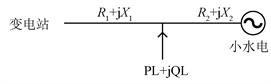Figure 1. Simplified schematic diagram of a small hydropower network

3.1. 小水电不参与发电

$\Delta P=\frac{{S}_{L}^{2}}{{U}_{N}^{2}}{R}_{1}$ (8)

3.2. 小水电参与发电

$\Delta {P}^{\prime }=\Delta {P}_{1}+\Delta {P}_{2}=\frac{{\left({S}_{L}-{S}_{水电}\right)}^{2}}{{U}_{N}^{2}}{R}_{1}+\frac{{S}_{水电}^{2}}{{U}_{N}^{2}}{R}_{2}$ (9)

$\Delta {P}_{add}=\Delta {P}^{\prime }-\Delta P=\frac{{S}_{水电}\left[{S}_{水电}\left({R}_{1}+{R}_{2}\right)-2{S}_{L}{R}_{1}\right]}{{U}_{N}^{2}}$ (10)

$\frac{{S}_{水电}}{{S}_{L}}>\frac{2{R}_{1}}{\left({R}_{1}+{R}_{2}\right)}$ (11)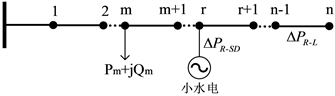Figure 2. Distribution line diagram with n nodes

$\Delta P=\Delta {P}_{R-L}+\Delta {P}_{R-SD}$ (12)

3.2.1. 节点之间的线损

$\Delta {P}_{R-L}=\Delta {P}_{0,1}+\Delta {P}_{1,2}+\cdots +\Delta {P}_{m,m+1}+\cdots +\Delta {P}_{r,r+1}+\cdots +\Delta {P}_{n-1,n}$ (13)

$\Delta {P}_{n-1,n}=\frac{{\left[{\left(-1\right)}^{{x}_{n}}{S}_{n}\right]}^{2}}{{U}_{N}^{2}}{R}_{n-1,n}$ (14)

$\Delta {P}_{n-2,n-1}=\frac{{\left[{\left(-1\right)}^{{x}_{n-1}}{S}_{n-1}+{\left(-1\right)}^{{x}_{n}}{S}_{n}\right]}^{2}}{{U}_{N}^{2}}{R}_{n-2,n-1}$ (15)

$\Delta {P}_{0,1}=\frac{{\left[{\left(-1\right)}^{{x}_{1}}{S}_{1}+{\left(-1\right)}^{{x}_{2}}{S}_{2}+\cdots +{\left(-1\right)}^{{x}_{n-1}}{S}_{n-1}+{\left(-1\right)}^{{x}_{n}}{S}_{n}\right]}^{2}}{{U}_{N}^{2}}{R}_{0,1}$ (16)

3.2.2. 小水电电源与配电线路之间的线损

$\Delta {P}_{R-SD}=\Delta {P}_{1}+\Delta {P}_{2}+\cdots +\Delta {P}_{r}+\cdots +\Delta {P}_{n}$ (17)

$\Delta {P}_{R-SD}=\underset{i=1}{\overset{n}{\sum }}{x}_{n}\frac{{S}_{n}^{2}}{{U}_{N}^{2}}{R}_{n}$ (18)

3.2.3. 某台小水电对配电线路总线损的贡献

$\frac{\partial \Delta {P}_{n-1,n}}{\partial {S}_{n}}={\left(-1\right)}^{{x}_{n}}\frac{2}{{U}_{N}^{2}}\left[{\left(-1\right)}^{{x}_{1}}{S}_{1}+{\left(-1\right)}^{{x}_{2}}{S}_{2}+\cdots +{\left(-1\right)}^{{x}_{n-1}}{S}_{n-1}+{\left(-1\right)}^{{x}_{n}}{S}_{n}\right]{R}_{0,1}$ (19)

$\frac{\partial \Delta {P}_{R-L}}{\partial {S}_{n}}=\frac{\partial \Delta {P}_{0,1}}{\partial {S}_{n}}+\frac{\partial \Delta {P}_{1,2}}{\partial {S}_{n}}+\cdots +\frac{\partial \Delta {P}_{n-1,n}}{\partial {S}_{n}}$ (20)

$\frac{\partial \Delta {P}_{R-L}}{\partial {S}_{n}}={\left(-1\right)}^{{x}_{n}}\frac{2}{{U}_{N}^{2}}\left\{\left[\underset{i=1}{\overset{n}{\sum }}{\left(-1\right)}^{{x}_{n}}{S}_{n}\right]{R}_{0,1}+\left[\underset{i=2}{\overset{n}{\sum }}{\left(-1\right)}^{{x}_{n}}{S}_{n}\right]{R}_{1,2}+\cdots +\left[{\left(-1\right)}^{{x}_{n}}{S}_{n}\right]{R}_{n-1,n}\right\}$ (21)

$\frac{\partial \Delta {P}_{R-L}}{\partial {S}_{n}}={\left(-1\right)}^{{x}_{n}}\frac{2}{{U}_{N}^{2}}\left\{{\left(-1\right)}^{{x}_{1}}{R}_{0,1}{S}_{1}+{\left(-1\right)}^{{x}_{2}}\left({R}_{0,1}+{R}_{1,2}\right){S}_{2}+\cdots +{\left(-1\right)}^{{x}_{n}}\left(\underset{i=1}{\overset{n}{\sum }}{R}_{n-1,n}\right){S}_{n}\right\}$ (22)

$\frac{\partial \Delta {P}_{R-SD}}{\partial {S}_{n}}=\underset{i=1}{\overset{n}{\sum }}2{x}_{n}\frac{{S}_{n}}{{U}_{N}^{2}}{R}_{n}$ (23)

$\frac{\partial \Delta P}{\partial {S}_{n}}=\frac{\partial \Delta {P}_{R-L}}{\partial {S}_{n}}+\frac{\partial \Delta {P}_{R-SD}}{\partial {S}_{n}}$ (24)

4. 降损措施

4.1. 优化配电线路区段导线截面

$\frac{\partial \Delta {P}_{n-1,n}}{\partial {R}_{n-1,n}}=\frac{{\left[{\left(-1\right)}^{{x}_{n}}{S}_{n}\right]}^{2}}{{U}_{N}^{2}}$ (25)

$\frac{\partial \Delta {P}_{n-2,n-1}}{\partial {R}_{n-2,n-1}}=\frac{{\left[{\left(-1\right)}^{{x}_{n-1}}{S}_{n-1}+{\left(-1\right)}^{{x}_{n}}{S}_{n}\right]}^{2}}{{U}_{N}^{2}}$ (26)

$\frac{\partial \Delta {P}_{0,1}}{\partial {R}_{0,1}}=\frac{{\left[{\left(-1\right)}^{{x}_{1}}{S}_{1}+{\left(-1\right)}^{{x}_{2}}{S}_{2}+\cdots +{\left(-1\right)}^{{x}_{n-1}}{S}_{n-1}+{\left(-1\right)}^{{x}_{n}}{S}_{n}\right]}^{2}}{{U}_{N}^{2}}$ (27)

$C=\frac{\left(1-k\right)Yf\Delta {P}_{s,s+1}}{xL}$(28)

4.2. 优化小水电接入位置

5. 算例分析

5.1. 枯水期与丰水期总线损计算Table 1. Branch parameters of distribution line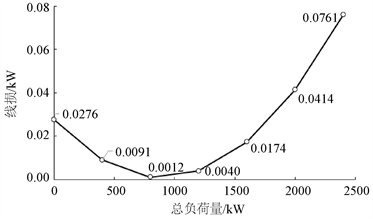(a) 枯水期负荷变化时配电线路总线损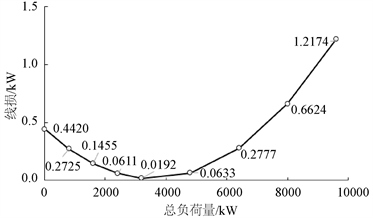(b) 丰水期负荷变化时配电线路总线损

Figure 3. Total line loss of distribution line when load changes in different seasons

5.2. 应用降损措施

5.2.1. 增大导线截面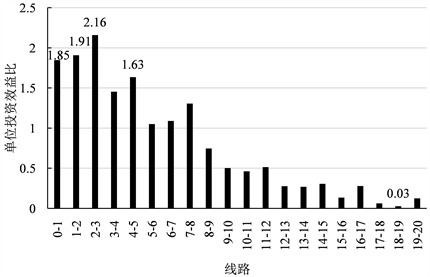Figure 4. Efficiency ratio per unit investment after changing wire resistance

5.2.2. 更换小水电接入位置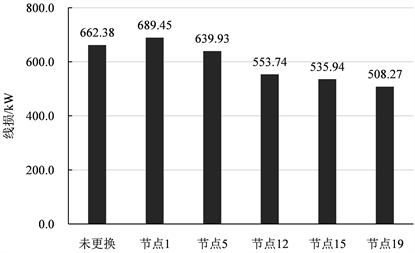Figure 5. Comparison of line loss after changing small hydropower to different positions

6. 结论

  刘丙江. 线损管理与节约用电[M]. 北京: 中国水利水电出版社, 2005.  姜富华, 杜孝忠. 我国小水电发展现状及存在的问题[J]. 中国农村水利水电, 2004(3): 82-83+86.  邹体峰, 王仲珏. 我国小水电开发建设中存在的问题及对策探讨[J]. 中国农村水利水电, 2007(2): 82-84.  彭智乐, 王星华, 彭显刚, 潘高峰. 基于区域同步特性的小水电出力随机建模及其准入容量评估[J]. 水电能源科学, 2015, 33(11): 171-175.  Elgerd, O.I. and Happ, H.H. (2007) Electric Energy Systems Theory: An Introduction. IEEE Transactions on Systems Man & Cybernetics, SMC-2, 296-297. https://doi.org/10.1109/TSMC.1972.4309116  史立山. 中国能源现状分析和可再生能源发展规划[J]. 可再生能源, 2004(5): 1-4.  Nadira, R., Wu, F.F., Maratukulam, D.J., Weber, E.P. and Thomas, C.L. (1990) Bulk Transmission System Loss Analysis. IEEE Transactions on Power Systems, 8, 405-416. https://doi.org/10.1109/59.260846  高久国. 探索小水电的综合管理新模式[D]: [硕士学位论文]. 杭州: 浙江大学, 2010.  郑华槟. 有小水电上网线路的线损考核[J]. 农村电气化, 2005(12): 46.  张海超. 基于唐山供电公司的线损分析及降损措施的研究[D]: [硕士学位论文]. 北京: 华北电力大学, 2013.  黄细琴. 小水电上网对配网线损影响的解决措施[J]. 农村电气化, 2018(6): 78.  陈玉波. 解决农村电网小水电并网线损增大实用方法研究[C]//云南电网公司、云南省电机工程学会. 2009年云南电力技术论坛论文集(优秀论文部分). 昆明: 云南省电机工程学会, 2009: 299-303.  何伟龙. 含多小水电的配电线路降损措施研究[D]: [硕士学位论文]. 广州: 广东工业大学, 2018.  谢云秋. 韶关中低压配电网线损分析及降损措施研究[D]: [硕士学位论文]. 广州: 华南理工大学, 2017.  韩伟光, 宋唯, 王学庆. 均方根电流法计算低压线损[J]. 煤炭技术, 1999, 18(6): 7-8.  高国梁. 分布式电源接入后的配电网降损及规划问题研究[D]: [硕士学位论文]. 济南: 山东大学, 2019.  汝绪丽. 一种含分布式电源的配电网线损计算方法[D]: [硕士学位论文]. 济南: 济南大学, 2019.  江木, 孙云超, 刘巨. 10 kV配电网理论线损计算方法及应用对比[J]. 供用电, 2019, 36(11): 78-83.  周有学. 配电网的线损理论计算及降损措施[D]: [硕士学位论文]. 重庆: 重庆大学, 2001.  樊华瑞. 多小水电接入型10 kV配电网电压控制策略研究[D]: [硕士学位论文]. 重庆: 重庆理工大学, 2018.